# Rasterization and Vectorization: How to Convert Data FormatsRasterization converts vectors into rasters. While vectorization transforms rasters in vectors. We explain how to go from one data model to the other.

### How is Rasterize Different From Vectorize?

Rasterization refers to converting vectors into rasters. While vectorization transforms rasters in vectors.

We know the two main data models and graphics are vector and raster data.

But how do you go from one data model to the other?

That is when you would use rasterization and vectorization. Learn how to do this for free with open source QGIS. But first for some basics:

READ MORE: GIS Spatial Data Types: Vector vs Raster

### What is a Vector?

Vector data are composed of vertices and paths. For example, the three types of vectors are points, polylines and polygons.

And we often store these points, lines and polygons in shapefiles, geodatabases and various other GIS file formats.

### What is a Raster?

Unlike vector data, rasters are made up of pixels or grid cells. Further to that, they are usually regularly spaced and square (but they don’t have to be) and often look pixellated.

Discrete rasters are categorical and have distinct values identifying each cell. For example a land cover raster might represent urban as the value 1 and forest as 2.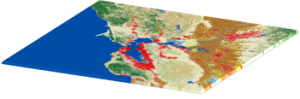Continuous rasters are grid cells with gradual changing data such as elevation, temperature or an aerial photograph. Continuous data is also known as non-discrete or surface data.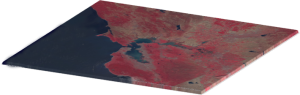READ MORE: 15 Free Satellite Imagery Data Sources

### Rasterization: Convert Vector to Raster

In this example, we are going to use the open source program QGIS.

Unlike graphic editing software, it expects your rasters and vectors to have a geographic coordinate system. But really it has similar functionality to Adobe Photoshop or GIMP that you can perform for rasterization and vectorization.

Let’s start with a simple vector line with a vertex at the start and end points.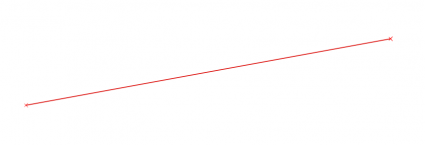Use the  Rasterize (Vector to Raster) Tool . The horizontal and vertical parameters will determine the output pixel size. After setting all the parameters, click RUN for rasterization.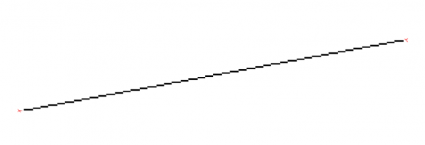Finally, the output will look like a gridded version of your vector. Depending on the pixel size you set, you may have to do some trial-and-error for better results.

### Vectorization: Convert Raster to Vector

Let’s go in reverse this time and take a discrete raster and convert it to vector.

When we have a raster data set like our buildings layer, it’s a matter of adding it to QGIS. Here’s what the raster buildings look like in the table of contents: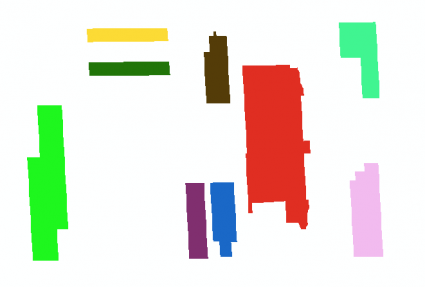QGIS comes loaded with algorithms and plugins for you. Further to this, other providers like GRASS, SAGA GIS and Orfeo toolbox brings additional firepower to the table. In this case, the  vectorization tool (v.to.vect)  in GRASS is the one you’re looking for.

Double-click the tool and select the raster that you want to convert to vector. From here, you’ll have to select the output of the raster to a point, line or polygon. After clicking RUN, the vectorized raster will appear in the table of contents.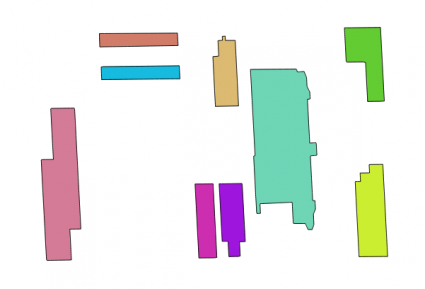As a reminder, vectorization creates all the necessary vertices and paths as shown with the building outlines. In a nutshell, this tool digitizes the raster for you converting it to a vector.

If you want to learn how to vectorize a continuous raster, this will take a bit of effort to reclassify cells essentially grouping them into discrete form. Using a temperature raster as an example, when you group values from [-37.8 to 0] as 0 and [0 to 37.7] as 1, you are turning it into a discrete raster. From here, you can follow these exact same steps for vectorization.

### Conclusion

For the most part, it’s a basic process to vectorize or rasterize images.

Rasterization refers to converting vectors into rasters. While vectorization transforms rasters in vectors.

These are the essential steps how to go from one data model to another.

Any questions? Let us know below.